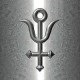# Creative days Invocation.RARE QUOTES

## Pythagorean symbol

1. The first four numbers symbolize the harmony of the spheres and the Cosmos as:
1. (1) Unity (Monad)
2. (2) Dyad – Power – Limit/Unlimited (peras/apeiron)
3. (3) Harmony (Triad)
4. (4) Kosmos (Tetrad).
2. The four rows add up to ten, which was unity of a higher order (The Dekad).
3. The Tetractys symbolizes the four elementsfire, air, water, and earth.
4. The Tetractys represented the organization of space:
1. the first row represented zero dimensions (a point)
2. the second row represented one dimension (a line of two points)
3. the third row represented two dimensions (a plane defined by a triangle of three points)
4. the fourth row represented three dimensions (a tetrahedron defined by four points)

https://en.wikipedia.org/wiki/Tetractys#Kabbalist_symbolView original post